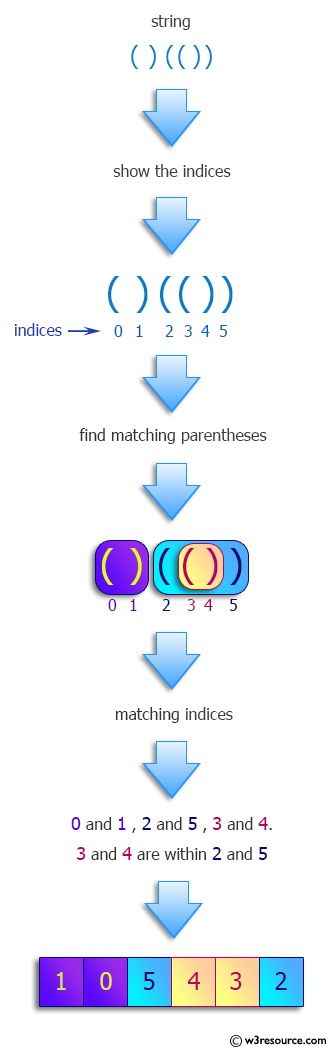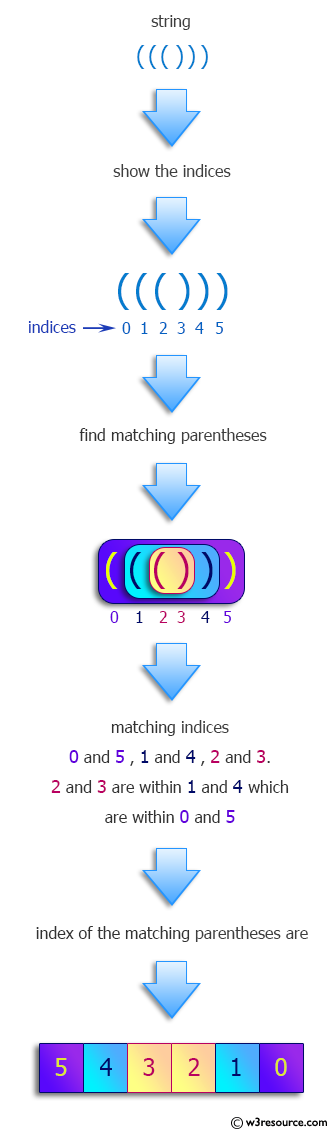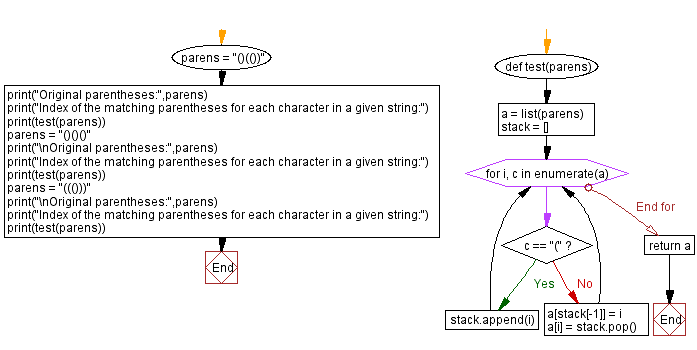﻿ Python: Find the index of the matching parentheses for each character in a given string - w3resource# Python: Find the index of the matching parentheses for each character in a given string

## Python Programming Puzzles: Exercise-84 with Solution

Write a Python program to find the index of the matching parentheses for each character in a given string.

```Input:
()(())
Output:
[1, 0, 5, 4, 3, 2]

Input:
()()()
Output:
[1, 0, 3, 2, 5, 4]

Input:
((()))
Output:
[5, 4, 3, 2, 1, 0]
```

Pictorial Presentation:Sample Solution:

Python Code:

``````#License: https://bit.ly/3oLErEI

def test(parens):
a = list(parens)
stack = []
for i, c in enumerate(a):
if c == "(":
stack.append(i)
else:
a[stack[-1]] = i
a[i] = stack.pop()
return a

parens = "()(())"
print("Original parentheses:",parens)
print("Index of the matching parentheses for each character in a given string:")
print(test(parens))
parens = "()()()"
print("\nOriginal parentheses:",parens)
print("Index of the matching parentheses for each character in a given string:")
print(test(parens))
parens = "((()))"
print("\nOriginal parentheses:",parens)
print("Index of the matching parentheses for each character in a given string:")
print(test(parens))
``````

Sample Output:

```Original parentheses: ()(())
Index of the matching parentheses for each character in a given string:
[1, 0, 5, 4, 3, 2]

Original parentheses: ()()()
Index of the matching parentheses for each character in a given string:
[1, 0, 3, 2, 5, 4]

Original parentheses: ((()))
Index of the matching parentheses for each character in a given string:
[5, 4, 3, 2, 1, 0]
```

Flowchart:## Visualize Python code execution:

The following tool visualize what the computer is doing step-by-step as it executes the said program:

Python Code Editor :

Have another way to solve this solution? Contribute your code (and comments) through Disqus.

What is the difficulty level of this exercise?

Test your Programming skills with w3resource's quiz.

﻿

## Python: Tips of the Day

Clamps num within the inclusive range specified by the boundary values x and y:

Example:

```def tips_clamp_num(num,x,y):
return max(min(num, max(x, y)), min(x, y))
print(tips_clamp_num(2, 4, 6))
print(tips_clamp_num(1, -1, -6))
```

Output:

```4
-1
```